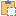#### Create Method (Document, XYZ, XYZ, XYZ, PointLoadType, SketchPlane)

Creates a new non-hosted point load within the project using data at point.

Namespace: Autodesk.Revit.DB.Structure
Assembly: RevitAPI (in RevitAPI.dll) Version: 16.0.0.0 (16.0.0.0)
Since: 2016

# Syntax

C#
``````public static PointLoad Create(
XYZ point,
XYZ forceVector,
XYZ momentVector,
SketchPlane plane
)``````
Visual Basic
``````Public Shared Function Create ( _
point As XYZ, _
forceVector As XYZ, _
momentVector As XYZ, _
plane As SketchPlane _
Visual C++
``````public:
XYZ^ point,
XYZ^ forceVector,
XYZ^ momentVector,
SketchPlane^ plane
)``````

#### Parameters

Type: Autodesk.Revit.DB Document
point
Type: Autodesk.Revit.DB XYZ
The position of point load, measured in decimal feet.
forceVector
Type: Autodesk.Revit.DB XYZ
The applied 3d force vector.
momentVector
Type: Autodesk.Revit.DB XYZ
The applied 3d moment vector.
symbol
The symbol of the PointLoad. Set a null reference ( Nothing in Visual Basic) to use default type.
plane
Type: Autodesk.Revit.DB SketchPlane
The work plane of the PointLoad. Set a null reference ( Nothing in Visual Basic) to use default plane.

#### Return Value

If successful, returns the newly created PointLoad, a null reference ( Nothing in Visual Basic) otherwise.

# ExamplesCopy C#
``````public PointLoad CreatePointLoad(Autodesk.Revit.DB.Document document)
{
//Define the location at which the PointLoad is applied.
XYZ point = new XYZ(0, 0, 4);
//Define the 3d force vector.
XYZ force = new XYZ(0, 0, -1);
//Define the 3d moment vector.
XYZ moment = new XYZ(0, 0, 0);

}``````Copy VB.NET
``````'Define the location at which the PointLoad is applied.
Dim point As New XYZ(0, 0, 4)
'Define the 3d force.
Dim force As New XYZ(0, 0, -1)
'Define the 3d moment.
Dim moment As New XYZ(0, 0, 0)